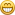symmetric spline wavefunction

Started by mogn, May 07, 2011, 09:33:58 am

mognMay 07, 2011, 09:33:58 am
In this wave function you have control over:

the line along which the wave is generated
the wavelength
the amplitude 1 and 2 third of the wavelength
the amplitude in the middle of the wave.

The wave has zero amplitude and gradient in the end points.
Uses a 6 order spline.

HetzenMay 07, 2011, 05:37:23 pm #1
Thanks Mogn. I always like to have a look at how you do things. I'm not sure how the math works with the dot product giving you the cos of the resultant angle, but I like it. It's one of those nodes I've never used, or found a use for before. But you've made this network easy to follow, and I can see this being very useful in the future. By my understanding, the modulo will give you parallel ramps in any direction defined by the top left constant vector.

Out of interest, how would you go about creating a vector direction from say an angle in a 360 arc defined by a constant scaler?

Thanks for sharing. Much appreciated.

Jon

DuneMay 08, 2011, 03:51:10 am #2
Thanks Mogn, very interesting. I'll check it out.

mognMay 08, 2011, 04:41:16 am #3 Last Edit: May 08, 2011, 04:45:43 am by mogn
The documentation of the dot function is partly wrong and not usable.
A better definition is: [A, B, C] dot [x, y, z] == [Ax, By, Cz]

Converting an arc scalar v to a vector:
Vector = [cos(v), 0, sin(v)]

joMay 09, 2011, 12:59:44 am #4
Hi Jon,

Quote from: Hetzen on May 07, 2011, 05:37:23 pm
Out of interest, how would you go about creating a vector direction from say an angle in a 360 arc defined by a constant scaler?

You can use the rotate function nodes for that. They rotate about the origin so if you need to rotate about another point you may need to do a bit more work.

Regards,

Jo

mognMay 09, 2011, 03:45:36 pm #5
The backside of the rotation function is that thr angle cannot be calculated by blue notes.

Before anybody discovers the errors in my calculations, I must go back to the drawingbord.

HetzenMay 11, 2011, 06:45:12 am #6
Quote from: jo on May 09, 2011, 12:59:44 am
Hi Jon,

Quote from: Hetzen on May 07, 2011, 05:37:23 pm
Out of interest, how would you go about creating a vector direction from say an angle in a 360 arc defined by a constant scaler?

You can use the rotate function nodes for that. They rotate about the origin so if you need to rotate about another point you may need to do a bit more work.

Regards,

Jo

Thanks Jo.

As Mogn has mentioned, the values are then locked in the Rotation node, so can't be used in further parts of the calculation, or modulated to animate for example.

Cheers

Jon

HetzenMay 11, 2011, 06:47:15 am #7
Quote from: mogn on May 08, 2011, 04:41:16 am
The documentation of the dot function is partly wrong and not usable.
A better definition is: [A, B, C] dot [x, y, z] == [Ax, By, Cz]

Converting an arc scalar v to a vector:
Vector = [cos(v), 0, sin(v)]

Thanks again Mogn. It really is that simple.

I'm slowly re-remembering my trig class at school.joMay 11, 2011, 07:37:25 am #8
Hi mogn,

Quote from: mogn on May 09, 2011, 03:45:36 pm
The backside of the rotation function is that thr angle cannot be calculated by blue notes.

Ah, right, of course. Sorry for the misdirection.

Regards,

Jo

neon22May 15, 2011, 09:49:47 pm #9
Mogn - can you put the wave shape modifier into this too. (That Hetzen, Dune and others have been working on in this thread:
http://forums.planetside.co.uk/index.php?topic=6111.0)
I.e. based on whether the sine is going up or down - push it out or back...

mognMay 17, 2011, 04:02:24 am #10 Last Edit: May 17, 2011, 04:04:16 am by mogn
If I correct my algorithm, such that the middle amplitude is fixed and the amplitude in a fixed point between 0 and the middle point
is specified, this arrangment can be changed in the following way:

subtract the mod value from the scaled axis giving a number N for each wave.
calculate x= mod(PI*(N*(N+1),1)*0.4
use x instead if the fixe point between 0 and 0.5

This will give a wave with fixed wavelengt fixed max amplitude, but each wave has a different form.

correcting my algorith involves solving two equations with two variables:

A*f(x   ) + B*G(x  ) = amplitude at x
A*f(0.5) + B*g(0.5) = amplitude at 0.5

where f(x) = ((1-x)*x)**2 *((1-x)**2 + x**2)
g(x) = (1-x)**3 * x**3

The wave function being A*f(s) + B*g(s) where s is running from 0 to 1.0

mognMay 18, 2011, 02:20:14 pm #11
The node network used to solve two equations in two unknown is:

mognMay 21, 2011, 08:06:15 am #12
Updated Wavefunction.

If you draw a line between the points X!,Y! and X2,Y2 and the lines crosses the axis in a point > 1/3 the wave
starts and ends with a valley (values less than zero). if the crossing point is less than 1/3 the middle points lies in a
valley.

neon22May 21, 2011, 05:37:45 pm #13
amazing skills !! I am astounded...

FYI - for ocean waves - Trochoids are a close match.
Trochoid math: